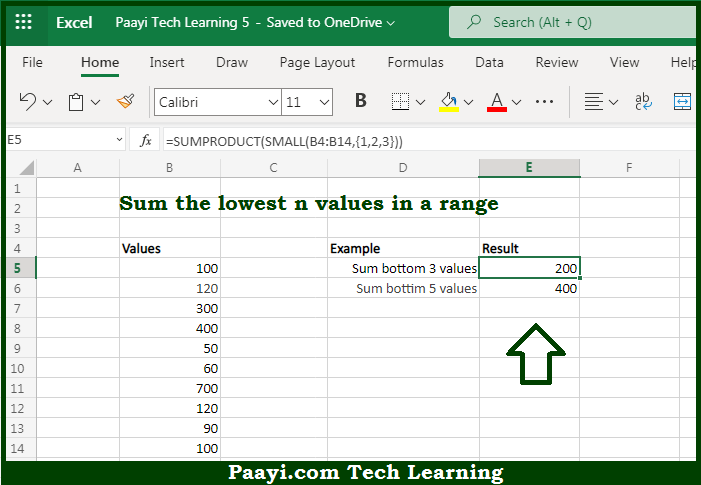# Learn How to SUM Bottom n Values in Microsoft Excel

Written by | 0 Comments | 476 Views

In this article, you will learn how to COUNT various things in Microsoft Excel using a single/combination(s) of functions. You will also know how to SUM Bottom n Values and see the generic formula.

SUM Bottom n Values in Microsoft Excel

The main purpose of this formula is to sum the lowest n values in a range. Here we will learn how to sum the bottom n values in Microsoft Excel. That implies, with the help of a formula based on the  SMALL an AGGREGATE function you can able to sum the lowest n values in a range. It should be noted that the generic form of the formula provided here, rng represents a range of cells that contain numeric values and n represents the number of lowest values to sum So, with the help of this formula, you can able to sum the bottom n values in the Microsoft Excel.

General Formula to SUM Bottom n Values

=SUMPRODUCT(SMALL(rng,{1,2,n}))

The Explanation for the SUM Bottom n ValuesSo we know that with the help of the given formula above you can able to sum the lowest n values in a range. Here we will learn how to sum the bottom n values in Microsoft Excel. As we know that the in the simplest form, the SMALL function will return the "nth smallest" value in a given range. But in case, you supply an array constant that is a constant in the form - 1,2,3, to SMALL function as the second argument, it will return an array of results instead of a single result So, with the help of this formula, you can able to sum the lowest n values in a range.